Contents >> Applied Mathematics >> Mathematical Statistics >> Treatment of Experiment Results >> Example

Treatment of experiment results - Example

Example

By means of a method of the least squares to pick up the 2-nd degree polynomial for the results of measurements of values of function y presented by the table at equidistant values of argument х :

 х у 0.1 1.316 0.2 1.633 0.3 1.976 0.4 2.378 0.5 2.723 0.6 3.201 0.7 3.572 0.8 4.051 0.9 4.500

S o l u t i o n .  As is known, a general view of the 2-nd degree polynomial: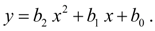Let's make system of Gauss’ normal equations: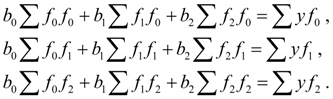Coefficients of system of these equations according to data of the table are equal to: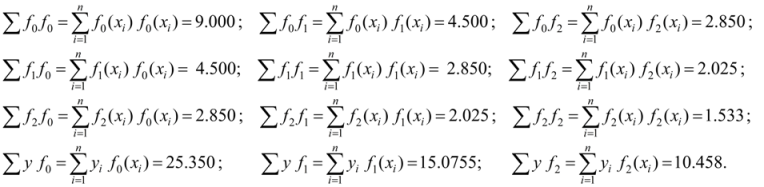After substitution of numerical values of coefficients the system of Gauss’normal equations becomes: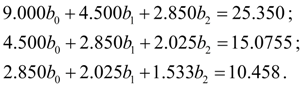Its solution: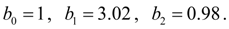Thus, the 2-nd degree required polynomial: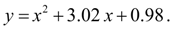< Previous Contents Next >